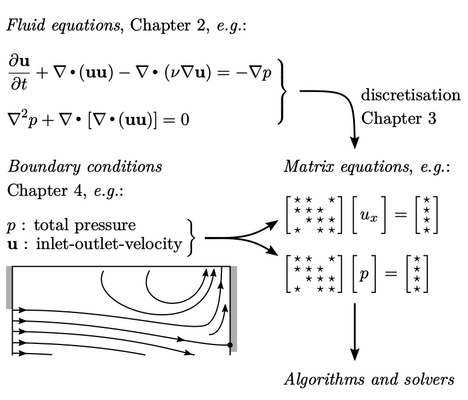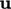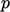## Chapter 5Algorithms and Solvers

“And you run, and you run to catch up with the sun but it’s sinking. Racing around to come up behind you again.”

Pink Floyd, Time (1973).

The previous chapters describe the components needed to build equations to solve problems in ﬂuid dynamics, which are listed below:

• governing equations and common models, see Chapter 2 ;
• numerical methods to create discrete sets of linear equations, as matrix equations, see Chapter 3 ;
• boundary condtitions, which are applied to the matrix equations, see Chapter 4 .

The ﬁnal component is to assemble the set of matrix equations and apply algorithms to:

• solve the set of linear equations described by each individual matrix equation;
• combine, or couple, solutions from multiple matrix equations to produce the overall solution.This chapter ﬁrst addresses the solution of individual matrix equations. It presents the most eﬀective algorithms for solving matrix equations encountered with the ﬁnite volume method.

The important requirements of these matrix solvers are robustness and eﬃciency, particularly for large simulations with many millions of cells. Solvers than accompany the ﬁnite volume method are generally iterative in nature, in which the solution converges towards a prescribed acceptable level of accuracy, or tolerance, over a number of iterations.

The principal algorithms that couple sets of matrix equations will then be presented. The coupling of mass and momentum conservation with equations forandis challenging, and so requires particular attention.

Notes on CFD: General Principles - Chapter 5 Algorithms and Solvers